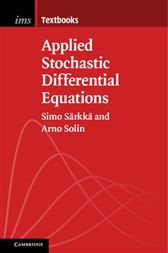# Applied Stochastic Differential Equations

## Series: Institute of Mathematical Statistics Textbooks (No. 10)30,94 €
###### ISBNs
• 9781316510087
• 9781108693431
• 9781108693448
Stochastic differential equations are differential equations whose solutions are stochastic processes. They exhibit appealing mathematical properties that are useful in modeling uncertainties and noisy phenomena in many disciplines. This book is motivated by applications of stochastic differential equations in target tracking and medical technology and, in particular, their use in methodologies such as filtering, smoothing, parameter estimation, and machine learning. It builds an intuitive hands-on understanding of what stochastic differential equations are all about, but also covers the essentials of Itô calculus, the central theorems in the field, and such approximation schemes as stochastic Runge–Kutta. Greater emphasis is given to solution methods than to analysis of theoretical properties of the equations. The book's practical approach assumes only prior understanding of ordinary differential equations. The numerous worked examples and end-of-chapter exercises include application-driven derivations and computational assignments. MATLAB/Octave source code is available for download, promoting hands-on work with the methods.

###### ISBNs
• 9781316510087
• 9781108693431
• 9781108693448

#### In The Press

'Stochastic differential equations have long been used by physicists and engineers, especially in filtering and prediction theory, and more recently have found increasing application in the life sciences, finance and an ever-increasing range of fields. The authors provide intended users with an intuitive, readable introduction and overview without going into technical mathematical details from the often-demanding theory of stochastic analysis, yet clearly pointing out the pitfalls that may arise if its distinctive differences are disregarded. A large part of the book deals with underlying ideas and methods, such as analytical, approximative and computational, which are illustrated through many insightful examples. Linear systems, especially with additive noise and Gaussian solutions, are emphasized, though nonlinear systems are not neglected, and a large number of useful results and formulas are given. The latter part of the book provides an up to date survey and comparison of filtering and parameter estimation methods with many representative algorithms, and culminates with their application to machine learning.' Peter Kloeden, Johann Wolfgang Goethe-Universität Frankfurt am Main

###### ISBNs
• 9781316510087
• 9781108693431
• 9781108693448